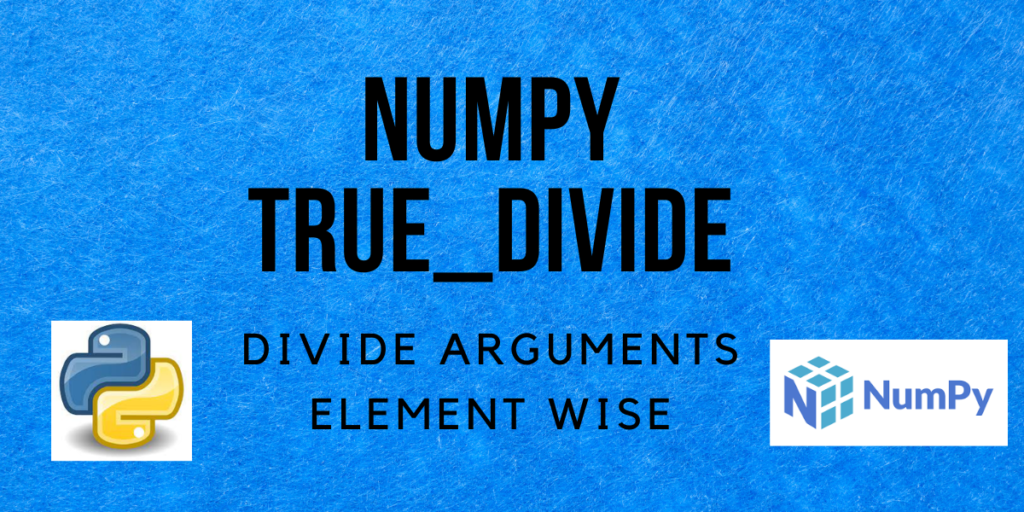# NumPy true_divide – Divide elements argument-wiseHello and welcome to this tutorial on Numpy true_divide. In this tutorial, we will be learning about the NumPy true_divide() method and also seeing a lot of examples regarding the same. So let us begin!

Also read: NumPy floor_divide – A Complete Guide

## What is NumPy true_divide?

`true_divide()` is a function in NumPy that divides elements from one array by elements in another array, element-wise and returns an array that contains the answer, i.e. quotient of each element-wise division.

If x1 and x2 are two arrays then `true_divide(x1, x2)` will perform element-wise division such that each element in x1 is divided by the corresponding element in x2 and store the result in a new array.

## Syntax of NumPy true_divide

Let us have a look at the syntax of the `true_divide()` function.

```numpy.true_divide(x1, x2, /, out=None, *, where=True, casting='same_kind', order='K', dtype=None, subok=True[, signature, extobj])
```

Returns:
An array containing the quotients of the element-wise division of x1 by x2.
If both the inputs are scalars, then the result is also scalar.

## Difference between floor_divide and true_divide

In Python, Numpy `floor_divide()` function performs the floor division of two arrays, element-wise. It is equivalent to using the `/` operator.

Whereas, the NumPy `true_divide()` performs division element-wise and is equivalent to using the `//` operator.

## Examples of NumPy true_divide

Lets now get right into the examples and understand how the `true_divide` method actually works.

### When both inputs are scalars

```import numpy as np

a = 18
b = 5
c = 6
# using the true_divide function to perform element-wise division
ans_1 = np.true_divide(a, b)
ans_2 = np.true_divide(a, c)

print("a =", a, "\nb =", b)
print("Result 1 =", ans_1)
print("Result 2 =", ans_2)
```

Output:

```a = 18
b = 5
Result 1 = 3.6
Result 2 = 3.0
```

Simple example where

```ans_1 = 18/5 = 3.6
ans_2 = 18/6 = 3
```

### When one input is a scalar and the other a 1-dimensional array

```import numpy as np

a = [2, 36, 10, 4, 20]
b = 5
# using the true_divide function to perform element-wise division
ans = np.true_divide(a, b)

print("a =", a, "\nb =", b)
print("Result =", ans)
```

Output:

```a = [2, 36, 10, 4, 20]
b = 5
Result = [0.4 7.2 2.  0.8 4. ]
```

Here, the scalar value is the divisor which divides each element in the dividend array as follows:

```ans = 2/5 = 0.4
ans = 36/5 = 7.2
ans = 10/5 = 2
ans = 4/5 = 0.8
ans = 20/5 = 4
```

### When both the input arrays are 1-dimensional

```import numpy as np

a = [5, 30, 12, 36, 11]
b = [2, 5, 6, 7, 10]
# using the true_divide function to perform element-wise division
ans = np.true_divide(a, b)

print("a =", a, "\nb =", b)
print("Result =", ans)
```

Output:

```a = [5, 30, 12, 36, 11]
b = [2, 5, 6, 7, 10]
Result = [2.5        6.         2.         5.14285714 1.1       ]
```

Here, each element in a is divided by the corresponding element in b and the output is calculated as:

```ans = a/b = 5/2 = 2.5
ans = a/b = 30/5 = 6
ans = a/b = 12/6 = 2
ans = a/b = 36/7 = 5.14285714
ans = a/b = 11/10 = 1.1
```

### When both the input arrays are 2-dimensional

```import numpy as np

a = [[25, 23], [12, 18]]
b = [[5, 6], [4, 5]]
# using the true_divide function to perform element-wise division
ans = np.true_divide(a, b)

print("a =", a, "\nb =", b)
print("Result =\n", ans)
```

Output:

```a = [[25, 23], [12, 18]]
b = [[5, 6], [4, 5]]
Result =
[[5.         3.83333333]
[3.         3.6       ]]
```

Similar to the above example,

```ans = a/b = 25/5 = 5
ans = a/b = 23/6 = 3.83333333

ans = a/b = 12/4 = 3
ans = a/b = 18/5 = 3.6
```

### Comparison of true_divide (//) and floor_divide (/)

```import numpy as np

a = [5, 30, 12, 36, 11]
b = [2, 5, 6, 7, 10]
# using the true_divide and floor_divide functions to perform element-wise division
ans_true_divide = np.true_divide(a, b)
ans_floor_divide = np.floor_divide(a, b)

print("a =", a, "\nb =", b)
print("Result of true divide =", ans_true_divide)
print("Result of floor divide =", ans_floor_divide)
```

Output:

```a = [5, 30, 12, 36, 11]
b = [2, 5, 6, 7, 10]
Result of true divide = [2.5        6.         2.         5.14285714 1.1       ]
Result of floor divide = [2 6 2 5 1]
```

The difference in the outputs here is that, in the output of floor division, the floor of the actual quotient is presented as the output as opposed to the true divide method where the actual quotients are included in the output.

## Conclusion

That’s all! In this tutorial, we learned about the Numpy true_divide method and practiced different types of examples using the same.